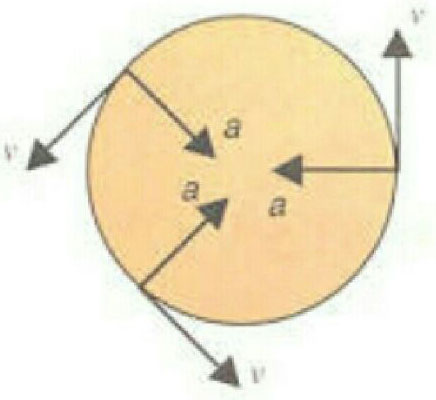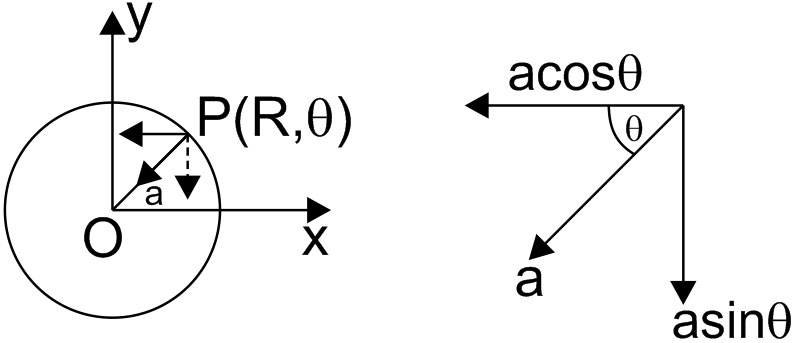# For a particle in uniform circular motion, the acceleration at a point on the circle of radius R is(Here is mesured by x-rays) Option 1) Option 2) Option 3) Option 4)

S Sabhrant Ambastha

As we learnt in

Centripetal acceleration -

When a body is moving in a uniform circular motion, a force is responsible to change direction of its velocity.This force acts towards the centre of circle and is called centripetal force.Acceleration produced by this force is centripetal acceleration.

- whereinFigure Shows Centripetal acceleration

For a  particle in uniform circular motionAcceleration

Option 1)

Incorrect

Option 2)

Incorrect

Option 3)

Incorrect

Option 4)

Correct

Exams
Articles
Questions